Next: What is simple/simplicial polytope? Up: Convex Polyhedron Previous: What is simplex?   Contents

## What is cube/hypercube/cross polytope?

A subset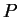of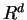is called a unit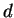-cube if it is the convex hull of all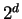points with components 0 or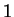. It has exactlyvertices and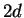facets. A cube or hypercube is a convex polytope which is isomorphic to the-cube for some.

A dual (see 2.4) of a cube is called a cross polytope.

Komei Fukuda 2004-08-26